## 5.7.1 Two-dimensional interpolation

In the case of two-dimensional interpolation, the type of interpolation to be used is set using the interpolate modifier to the set samples command, and may be changed at any time after the interpolation function has been created. The options available are nearest neighbor interpolation – which is the two-dimensional equivalent of stepwise interpolation, inverse square interpolation – which returns a weighted average of the supplied data points, using the inverse squares of their distances from the requested point in argument space as weights, and Monaghan Lattanzio interpolation, which uses the weighting function (Monaghan & Lattanzio 1985)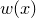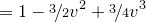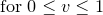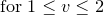where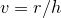for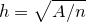,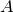is the product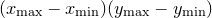and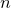is the number of input datapoints. These are selected as follows:

set samples interpolate nearestNeighbor
set samples interpolate inverseSquare
set samples interpolate monaghanLattanzio


set samples interpolate inverseSquare

Finally, data can be imported from graphical images in bitmap (.bmp) format to produce a function of two arguments returning a value in the range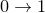which represents the data in one of the image’s three color channels. The two arguments are the horizontal and vertical position within the bitmap image, as measured in pixels. This is done using syntax of the form:
interpolate 2d bmp_b blue() 'myImg.bmp'